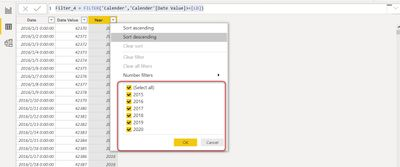cancel
Showing results for
Did you mean:Anonymous
Not applicable

## Power BI Desktop Filter Calendar Issue

Hi,

I enconter Power BI issue with Filter function.

1st Step: I try to add a Calendar table:

Calender = CALENDAR(DATE(2015,1,1),DATE(2020,12,31))

2nd Step: I filter the current year using below measure. and it works very well:
Filter_3 = FILTER('Calender','Calender'[Date Value]>=CALCULATE(MIN('Calender'[Date Value]),FILTER('Calender','Calender'[Year]=YEAR(NOW()))))Then I try to move above red color measure to a new measure:
LD = CALCULATE(MIN('Calender'[Date Value]),FILTER('Calender','Calender'[Year]=YEAR(NOW())))

and I create another table use ：
Filter_4 = FILTER('Calender','Calender'[Date Value]>=[LD])

but the new table can not applied any filter and showed all the calendar table:I don't know why this issue happen since I just splite a long measure into two portion. Anyone can help me to fix the problem? is this a Pow
3 REPLIES 3Solution Sage

Not sure what is the requirement here. However the issue is FILTER('Calender','Calender'[Date Value] . Filter as Calculated Column start a new Row Context. So basically it is getting evaluated for each row of base table.

Also, Filter returns a Table. So, you are trying to insert a Table for each row of Calendar table as  Column that is not allowed. To verify this, you can see below.

`Filter_3 = COUNTROWS(FILTER('Calender','Calender'[Date Value]>=CALCULATE(MIN('Calender'[Date Value]),FILTER('Calender','Calender'[Year]=YEAR(NOW())))))`

In Filter_4 - You are creating a Table using Filter that is perfectly fine.

Thanks
Ankit Jain

Do Mark it as solution if the response resolved your problem. Do like the response if it seems good and helpful.Anonymous
Not applicable

Dear Anki,

my problem is filter_3 works very well.

`Filter_3 = FILTER('Calender','Calender'[Date Value]>=CALCULATE(MIN('Calender'[Date Value]),FILTER('Calender','Calender'[Year]=YEAR(NOW()))))`
but it is too long. I try to copy part of above measure to create a new one and name it as LD:
`LD = CALCULATE(MIN('Calender'[Date Value]),FILTER('Calender','Calender'[Year]=YEAR(NOW())))`
and create new Filter_4 using below code:
`Filter_4 = FILTER('Calender','Calender'[Date Value]>=[LD])`
Since these two code should be same. I expect the Same result of Filter_3 and Filter_4. But Filter_4 table show all of the orginal Calender table, which means it do not aplly any filter that I set of "LD".

The orginal Calender table include: Year of "2015" to "2020".
I would like filter the year of current year and after current year:
the Filter_3 works very well, showing year of "2019" and "2020"
but Filter_4 shows all of them: Year of "2015" to "2020".

I can not understand what is the problem of these two almost "same" filter?Solution Sage

Hi - I can't tell exact reason why but to me it seems using this way in Filter 4, Measure LD is returning blank and hence you getting all the values. For your requirement, you can simply use below.

`Table 2 = FILTER(Calender,YEAR(Calender[Date]) >= YEAR(NOW()))`

Thanks
Ankit Jain

Do Mark it as solution if the response resolved your problem. Do like the response if it seems good and helpful.